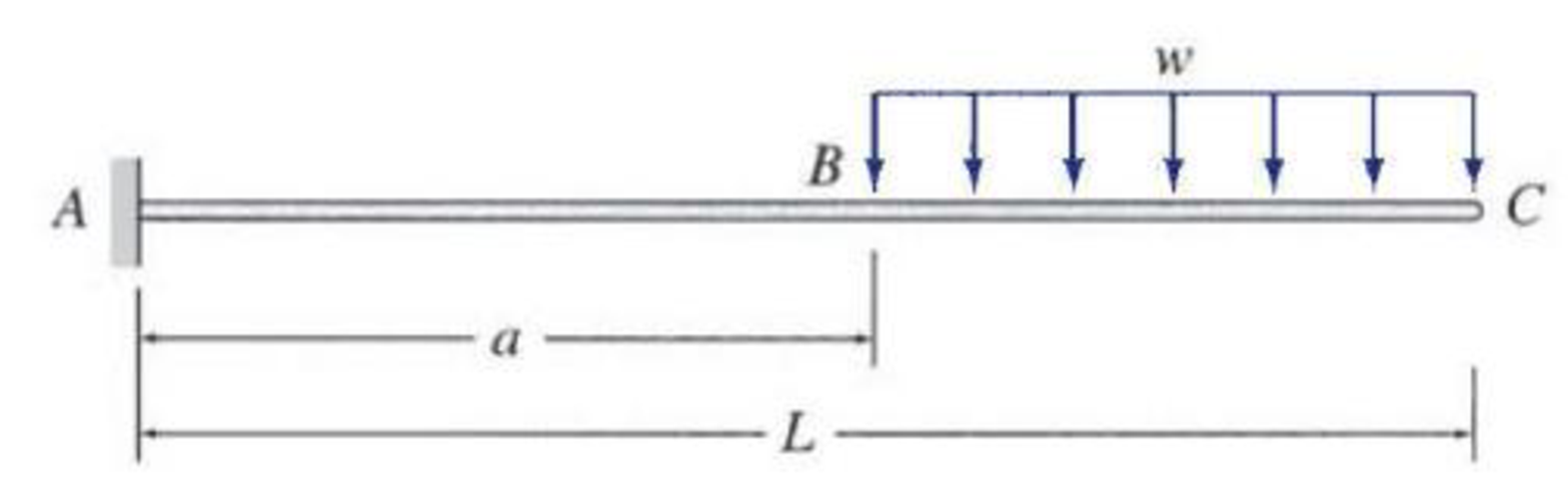# Determine the equations for slope and deflection of the beam shown by the direct integration method. EI = constant. FIG. P6.3

#### Solutions

Chapter
Section
Chapter 6, Problem 3P
Textbook Problem
144 views

## Determine the equations for slope and deflection of the beam shown by the direct integration method. EI = constant.FIG. P6.3

To determine

Find the equations for slope and deflection of the beam using direct integration method.

### Explanation of Solution

Calculation:

Draw the free body diagram of the beam as in Figure (1).

Refer Figure (1),

Find the reaction at support A.

Apply vertical equilibrium along y-axis.

Consider upward force as positive.

ΣFy=0Ayw(La)=0Ay=w(La)

Find the moment at A.

Consider anticlockwise moment as positive.

0=MAw×(La)×[(La)2+a]MA=w(La)[(La+2a2)]MA=w(La)(L+a2)MA=w2(L2a2)

Segment AB: 0xa.

Consider a section X1X1 in the segment AB at a distance of x from A.

Sketch the free body diagram when section X1X1 consider in the segment AB as shown in Figure 2.

Refer Figure (2),

Take the moment at section X1X1 .

M=w2(L2a2)+w×(La)(x)

Write the equation for MEI.

d2ydx2=MEI=1EI[w2(L2a2)+w(La)x]        (1)

Find the equation for slope (θ).

Integrate Equation (1) with respect to x.

dydx=θ=MEIdxθ=w2(L2a2)+w×(La)(x)EIdxEIθ=w2(L2a2)x+w×(La)(x22)+C1        (2)

Find the equation for deflection (θ).

Integrate again Equation (2) with respect to x.

EIy=w2(L2a2)x22+w×(La)(x36)+C1x+C2        (3)

Find the integration constants C1andC2:

Apply boundary conditions in Equation (2):

At x=0 and y=0.

0=M×022EI+C1×0+C2C2=0

Apply boundary conditions in Equation (1):

At x=0 and θ=0.

0=w2(L2a2)×0+w×(La)(022)+C1C1=0

Find the equation for slope of segment AB.

Substitute 0 for C1 in Equation (2).

EIθ=w2(L2a2)x+w×(La)(x22)+0EIθ=wx2(L2a2)+wx22(La)θ=wx2EI[a2L2+(La)x]

Thus, the equation for slope of segment AB is wx2EI[a2L2+(La)x]_.

Find the equation for deflection of segment AB.

Substitute 0 for C1 and 0 for C2 in Equation (3).

EIy=w2(L2a2)x22+w×(La)(x36)+0×x+0EIy=w2(L2a2)x22+w×(La)(x36)EIy=wx22(L2a22)+wx36(La)y=wx22EI(a2L22+(La)x3)

Thus, the equation for deflection is wx22EI(a2L22+(La)x3)_.

Segment BC: axL.

Consider a section X2X2 in the segment BC at a distance of x from A.

Sketch the free body diagram when section X2X2 consider segment BC as shown in Figure 3.

Refer Figure 3.

Write the equation for bending moment at section X2X2.

M=w2(L2a2)+w×(La)(x)w2(xa)2

Write the equation for MEI.

d2ydx2=MEI=1EI[w2(L2a2)+w×(La)(x)w2(xa)2]        (4)

Write the equation for slope.

Integrate Equation (4) with respect to x.

dydx=θ=MEIdxθ=[w2(L2a2)+w×(La)(x)w2(xa)2]EIdxEIθ=w2(L2a2)x+w×(La)(x22)w2(x33+a2xax2)+C3        (5)

Write the equation for deflection

### Still sussing out bartleby?

Check out a sample textbook solution.

See a sample solution

#### The Solution to Your Study Problems

Bartleby provides explanations to thousands of textbook problems written by our experts, many with advanced degrees!

Get Started

Find more solutions based on key concepts
What are the SI and U.S. Customary units for power?

Engineering Fundamentals: An Introduction to Engineering (MindTap Course List)

Use an elastic analysis and determine the maximum load per inch of weld.

Steel Design (Activate Learning with these NEW titles from Engineering!)

Describe the strategy of acceptance.

Management Of Information Security

Define the term slip.

Electric Motor Control

Linux is proprietary software.

Enhanced Discovering Computers 2017 (Shelly Cashman Series) (MindTap Course List)

What requirement does HIPAA institute to safeguard patient privacy?

Principles of Information Systems (MindTap Course List)

What is the difference between a context diagram d diagram 0? Which symbol is nor used in a context diagram?

Systems Analysis and Design (Shelly Cashman Series) (MindTap Course List)

Calculate tailstock setover for a 13.5" part with a TPF of 0.27.

Precision Machining Technology (MindTap Course List)

What is the maximum width of the cover pass? Why?

Welding: Principles and Applications (MindTap Course List)

If your motherboard supports ECC DDR3 memory, can you substitute non-ECC DDR3 memory?

A+ Guide to Hardware (Standalone Book) (MindTap Course List)